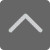## 爱智康资讯站

### 课程咨询: 4000-121-121

• 全国课程在线咨询
• 咨询热线：4000-121-121
• 登录手机官网

• 关注微信公众号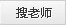# 期中备考初中数学公式：平方差公式

2020-10-22 17:53:05 　来源：本站原创

## 学而思・爱智康1对1/8人班 帮助孩子解决知识难点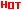*2470人已预约，首课体验不满意，可全额退费。

― ― 报名课程可获得 ― ―

公式运用

可用于某些分母含有根号的分式：

1/(3-4倍根号2)化简：

1×(3+4倍根号2)/(3-4倍根号2)^2;=(3+4倍根号2)/(9-32)=(3+4倍根号2)/-23

[解方程]

x^2-y^2=1991

[思路分析]

利用平方差公式求解

[解题过程]

x^2-y^2=1991

(x+y)(x-y)=1991

因为1991可以分成1×1991，11×181

所以如果x+y=1991，x-y=1，解得x=996，y=995

如果x+y=181，x-y=11，x=96，y=85同时也可以是负数

所以解有x=996，y=995，或x=996，y=-995，或x=-996，y=995或x=-996，y=-995

或x=96，y=85，或x=96，y=-85或x=-96，y=85或x=-96，y=-85

有时应注意加减的过程。

常见错误

平方差公式中常见错误有：

①学生难于跳出原有的定式思维，如典型错误;(错因：在公式的基础上类推，随意“创造”)

②混淆公式;

③运算结果中符号错误;

④变式应用难以掌握。

三角平方差公式

三角函数公式中，有一组公式被称为三角平方差公式：

(sinA)^2-(sinB)^2=(cosB)^2-(cosA)^2=sin(A+B)sin(A-B)

(cosA)^2-(sinB)^2=(cosB)^2-(sinA)^2=cos(A+B)sin(A-B)

这组公式是化积公式的一种，由于酷似平方差公式而得名，主要用于解三角形。

注意事项

1、公式的左边是个两项式的积，有一项是完全相同的。

2、右边的结果是乘式中两项的平方差，相同项的平方减去相反项的平方。

3、公式中的a.b可以是具体的数，也可以是单项式或多项式。

例题

一，利用公式计算

(1)103×97

解：(100+3)×(100-3)

=(100)^2-(3)^2

=100×100-3×3

=10000-9

=9991

(2)(5+6x)(5-6x)

解：5^2-(6x)^2

=25-36x^2

对数学掌握不是很好的同学，老师在这里给同学们推荐数学期中真卷冲刺课程，希望同学们考试顺利--》点击查看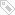标签：　期中数学备考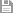保存　｜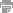打印　｜关闭